×#### Thank you for registering.

One of our academic counsellors will contact you within 1 working day.

Click to Chat

1800-1023-196

+91-120-4616500

CART 0

• 0

MY CART (5)

Use Coupon: CART20 and get 20% off on all online Study Material

ITEM
DETAILS
MRP
DISCOUNT
FINAL PRICE
Total Price: Rs.

There are no items in this cart.
Continue Shopping• Complete JEE Main/Advanced Course and Test Series
• OFFERED PRICE: Rs. 15,900
• View Details

```Chapter 18 Symmetry Exercise 18.3

Question: 1

Give the order of rotational symmetry for each of the following figures when rotated about the marked point (x):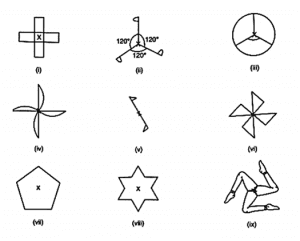Solution:

(i) The given figure has its rotational symmetry as 4.

(ii) The given figure has its rotational symmetry as 3.

(iii) The given figure has its rotational symmetry as 3.

(iv) The given figure has its rotational symmetry as 4.

(v) The given figure has its rotational symmetry as 2.

(vi) The given figure has its rotational symmetry as 4.

(vii) The given figure has its rotational symmetry as 5.

(viii) The given figure has its rotational symmetry as 6.

(ix) The given figure has its rotational symmetry as 3.

Question: 2

Name any two figures that have both line symmetry and rotational symmetry.

Solution:

An equilateral triangle and a square have both lines of symmetry and rotational symmetry.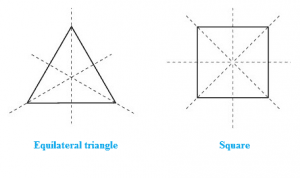Question: 3

Give an example of a figure that has a line of symmetry but does not have rotational symmetry.

Solution:

A semicircle and an isosceles triangle have a line of symmetry but do not have rotational symmetry.Question: 4

Give an example of a geometrical figure which has neither a line of symmetry nor a rotational symmetry.

Solution:

A scalene triangle has neither a line of symmetry nor a rotational symmetry.Question: 5

Give an example of a letter of the English alphabet which has

(i) No line of symmetry

(ii) Rotational symmetry of order 2.

Solution:

(i) The letter of the English alphabet which has no line of symmetry is Z.

(ii) The letter of the English alphabet which has rotational symmetry of order 2 is N.

Question: 6

What is the line of symmetry of a semi-circle? Does it have rotational symmetry?

Solution:

A semicircle (half of a circle) has only one line of symmetry. In the figure, there is one line of symmetry. The figure is symmetric along the perpendicular bisector I of the diameter XY.

A semi-circle does not have any rotational symmetry.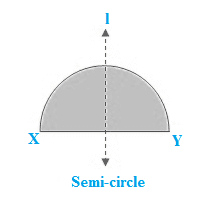Question: 7

Draw, whenever possible, a rough sketch of

(i) a triangle with both line and rotational symmetries.

(ii) a triangle with only line symmetry and no rotational symmetry.

(iii) a quadrilateral with a rotational symmetry but not a line of symmetry.

(iv) a quadrilateral with line symmetry but not a rotational symmetry.

Solution:

(i) An equilateral triangle has 3 lines of symmetry and a rotational symmetry of order 3.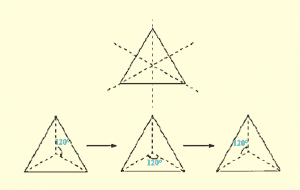(ii) An isosceles triangle has only 1 line of symmetry and no rotational symmetry.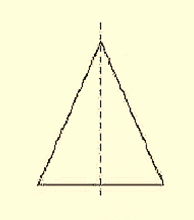(iii) A parallelogram is a quadrilateral which has no line of symmetry but a rotational symmetry of order 2.(iv) A kite is a quadrilateral which has only one line of symmetry and no rotational symmetry.Question: 8

Fill in the blanks:

Figures
Centre of rotation
Order of rotation
Angle of rotation

Square

Rectangle

Rhombus

Equilateral triangle

Regular hexagon

Circle

Semi-circle

Solution:

Figures
Centre of rotation
Order of rotation
Angle of rotation  (°)

Square

Point of intersection of the line segments joining the mid-points of opposite sides.

4
90

Rectangle

Point of intersection of the line segments joining the mid-points of opposite sides

2
180

Rhombus

Point of intersection of diagonals

2
180

Equilateral triangle

Point of intersection of angle bisectors i.e, centroid

3
120

Regular hexagon

Centre of the hexagon

6
60

Circle

Centre of the circle

Unlimited
Any angle

Semi-circle

Nil

NIL
Nil

Question: 9

Fill in the blanks:

English alphabet Letter
Line Symmetry
Number of Lines of symmetry
Rotational Symmetry
Order of rotational Symmetry

Z
Nil
0
YES
2

S
–
–
–
–

H
YES
–
YES
–

O
YES
–
YES
–

E
YES
–
–
–

N
–
–
YES
–

C
–
–
–
–

Solution:

English alphabet Letter
Line Symmetry
Number of Lines of symmetry
Rotational Symmetry
Order of rotational Symmetry

Z
NO
0
YES
2

S
NO
0
YES
2

H
YES
2
YES
2

O
YES
4
YES
2

E
YES
1
NO
0

N
NO
0
YES
2

C
YES
1
NO
0

```### Course Features

• 728 Video Lectures
• Revision Notes
• Previous Year Papers
• Mind Map
• Study Planner
• NCERT Solutions
• Discussion Forum
• Test paper with Video Solution